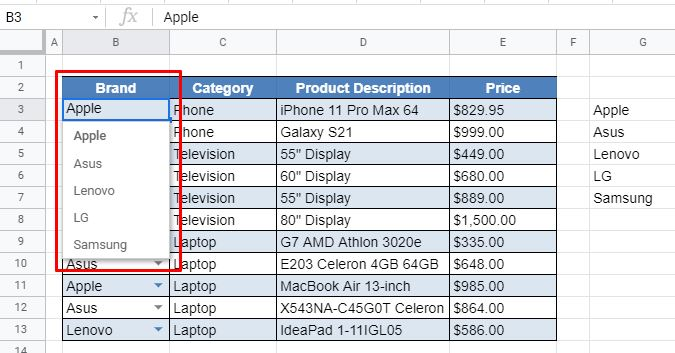# Sort / Alphabetize a Drop-Down List in Excel & Google Sheets

This tutorial will demonstrate how to alphabetize a drop-down list in Excel and Google Sheets.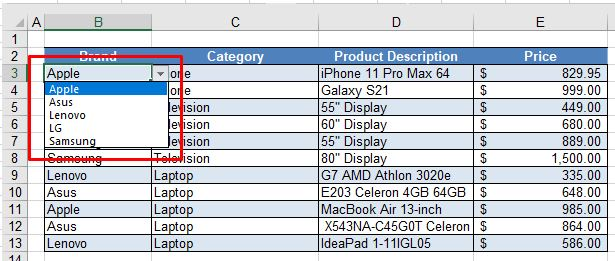When you enter repetitive data into Excel, it can sometimes be useful to have a drop-down list of options to select from. A powerful new feature of Excel 365 is the ability to sort data and only show unique data in a list due to a new feature called Dynamic Array Functions.

To obtain a drop-down list of unique values sorted into alphabetical order, use two of these new functions, namely the UNIQUE and SORT Functions. You can then use Data Validation to create a drop-down list from the data returned by these functions.

Note: It’s also possible to sort alphabetically using VBA.

## The SORT Function

The SORT Function allows you to sort a list of data into alphabetical order.

In a blank cell to the right of the data, type the following formula:

``=SORT(B3:B13)``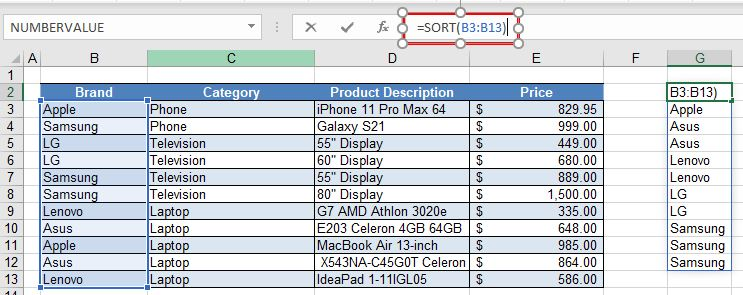When you press ENTER, or click the check mark to enter the formula into Excel, a list of sorted values from the selected range will appear beneath the cell where you entered the formula. This is known as the “spill range.”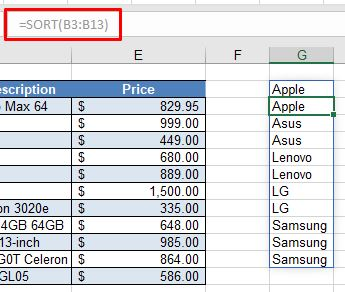The spill range automatically outputs all the unique values that are contained in the selected range. Notice that in the formula bar, the formula is grayed out in this spill range due to the fact that it is a Dynamic Array Formula. If you were to delete the formula in cell G2 for example, then the spill range would be cleared as well. The spill range is identifiable by the thin blue line that appears around it.

## The UNIQUE Function

The UNIQUE Function is another Dynamic Array Function that allows you to extract unique values from a list.

In a blank cell to the right of the data, type the following formula:

``=UNIQUE(B3:B13)``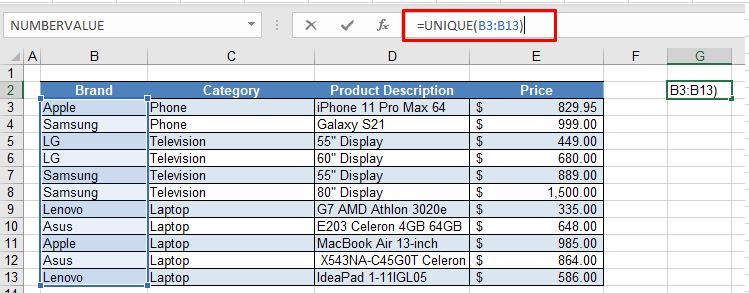As with the SORT Function, as soon as you press the ENTER key, the UNIQUE Function will spill over to the spill range and fill in the column below the cell where you have entered the formula. The list will only show unique values from the original selected range and as it is a Dynamic Array Formula, you cannot amend or alter the formula in this spill range.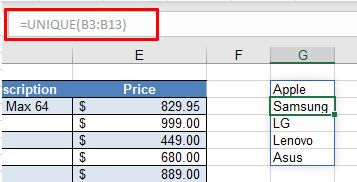## Combine the SORT and UNIQUE Functions

By combining the SORT and UNIQUE Functions together, you can obtain a list that only shows unique values and is sorted into alphabetical order.

``=SORT(UNIQUE((B3:B13)))``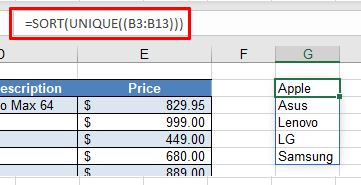Note: It does not matter in which order you nest the functions; you could also use the formula =UNIQUE(SORT(B3:B13)).

## Create the Drop-Down List

You can now use this range of cells to create a drop-down list to select from using Data Validation.

1. Select the range of cells where you wish the drop-down list to appear, and then in the Ribbon, select Data > Data Validation.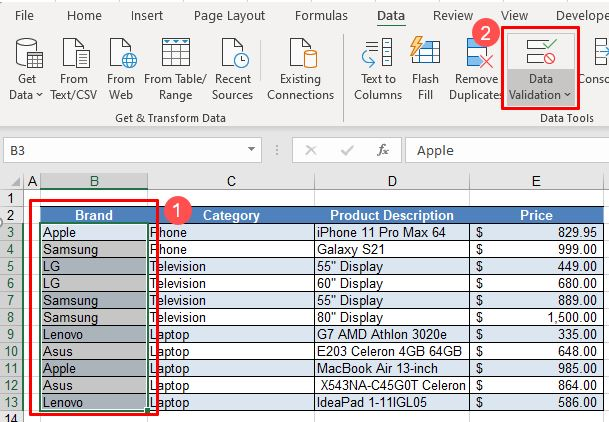1. Now select List from the Allow list, then type the formula for the Source of the list.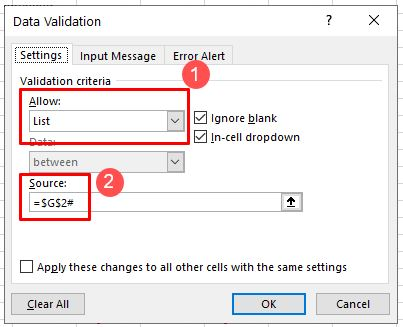It is necessary to put the hashtag (#) after the formula to let Excel know that you require the entire spill range and not just the value in the individual cell (e.g., G2).

1. Click OK to create the sorted drop-down list in the selected range.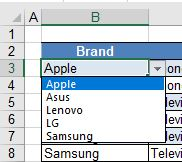## Alphabetize a Drop-Down List in Google Sheets

The SORT and UNIQUE Functions work the same way in Google Sheets as they do in Excel.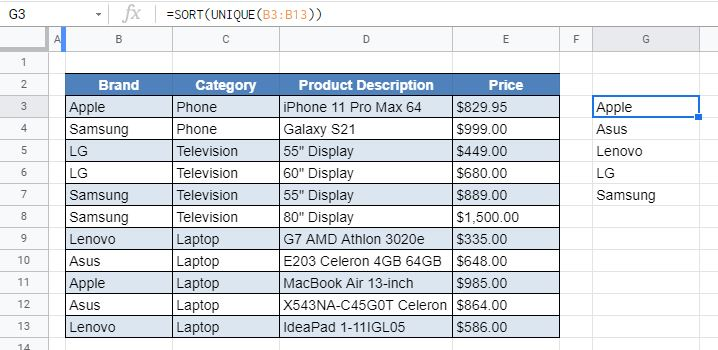1. To create the drop-down list, highlight the range of cells that will contain the drop-down list, and then in the Menu, select Data validation.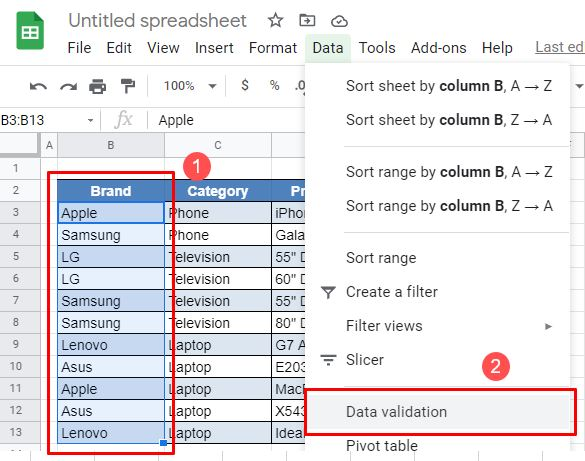1. While the cell range to contain the drop-down lists will automatically be filled in from the selection above, you need to enter the Criteria. List from a range is automatically selected, and then enter the entire cell range for the criteria list (e.g., G3: G7). The hashtag functionality that Excel uses does not exist in Google Sheets.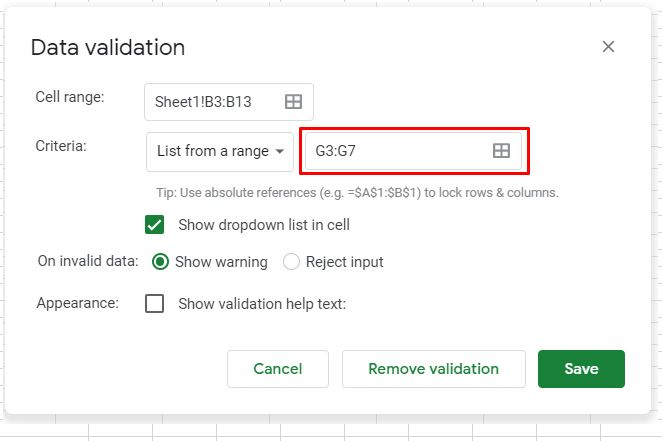1. Click Save to insert the sorted drop-down list into the Google spreadsheet.